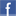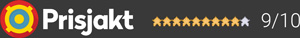#### Inga produkter tillagda!

Lägg en produkt i varukorgen så ser det lite roligare ut här ;)

#### Varukorg

0 kr# VincentPHO-200

1 490 krVälj utförande
1 490 kr
1 490 kr
Lagervara (Leverans 2-5 dagar)
Fri frakt!

## Fler bilder

Phono-Preamplifier for connecting your turntable to a line input of your amplifier. The low-noise phono-preamp is suitable for MM and MC cartridges. In order to avoid any humming noise an external power supply is used.

Technical Specifications
Frequency Response: 10 Hz – 20 kHz +/- 0.5 dB; 10 Hz – 50 kHz +/- 2 dB
Input Sensitivity MM: 58 mV, MC: 6,8 mV
Signar-Noise ratio: MM: >84 dB, MC: >71dB
Input Impedance MM: 47 kOhm, MC: 100 Ohm
Amplification factor: MM: 40 dB, MC: 100 dB
Inputs: 1 x Stereo RCA
Outputs: 1 x Stereo RCA
Power Supply: 12 V DC, 200 mA
Colour: Black or Silver
Weight: 1 kg
Dimensions (W x H x D): 115x55x130 mm

Garanti i 24 månaderDela på Facebook

#### Frågor? Ring 031-241600eller maila //<![CDATA[ var l=new Array(); l = '>'; l = 'a'; l = '/'; l = '<'; l = '|109'; l = '|111'; l = '|99'; l = '|46'; l = '|110'; l = '|114'; l = '|97'; l = '|108'; l = '|108'; l = '|97'; l = '|107'; l = '|100'; l = '|117'; l = '|106'; l = '|108'; l = '|64'; l = '|111'; l = '|102'; l = '|110'; l = '|105'; l = '>'; l = '"'; l = '|109'; l = '|111'; l = '|99'; l = '|46'; l = '|110'; l = '|114'; l = '|97'; l = '|108'; l = '|108'; l = '|97'; l = '|107'; l = '|100'; l = '|117'; l = '|106'; l = '|108'; l = '|64'; l = '|111'; l = '|102'; l = '|110'; l = '|105'; l = ':'; l = 'o'; l = 't'; l = 'l'; l = 'i'; l = 'a'; l = 'm'; l = '"'; l = '='; l = 'f'; l = 'e'; l = 'r'; l = 'h'; l = ' '; l = 'a'; l = '<'; for (var i = l.length-1; i >= 0; i=i-1) { if (l[i].substring(0, 1) === '|') document.write("&#"+unescape(l[i].substring(1))+";"); else document.write(unescape(l[i])); } //]]>